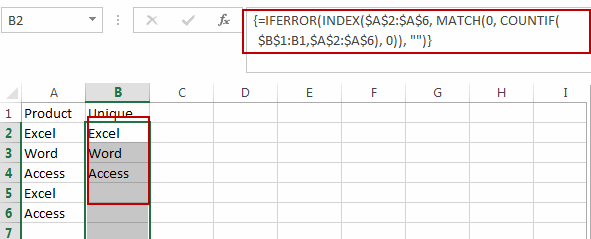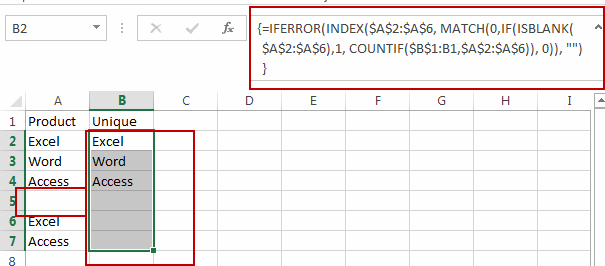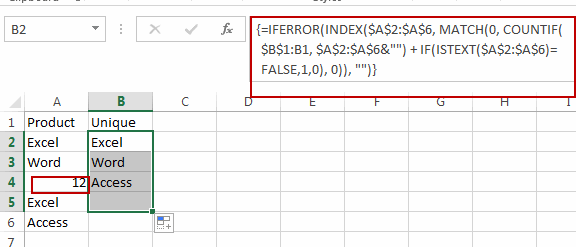# How to Extract a Unique Distinct List from a Column in Excel

This post will guide you how to extract a unique distinct list from two or more columns in Excel. How do I get a list of distinct values in multiple columns with a formula in Excel. How to create a list of unique values from a range of cells using VBA Code in Excel.

## Extract Unique Values in Column Using Formula

Assuming that you have a list of data in range A1:A6, and the list has duplicate values, and you want to extract a unique distinct list from a range of cells in a column. How to do it. You can use an array formula based on the IFERROR function, the INDEX function, the MATCH function and the COUNTIF function to achieve the result. Like this:

`=IFERROR(INDEX(\$A\$2:\$A\$6, MATCH(0, COUNTIF(\$B\$1:B1,\$A\$2:\$A\$6), 0)), "")`

Type this formula into cell B2, and press Ctrl + Shift + Enter key on your keyboard to change it as array formula, and then drag the AutoFill Handle down to other cells to extract all unique values from a range A1:A6.If you want to extract Unique Values from a range in one column ignoring blank cells, you can use the following array formula to achieve it. Like this:

`=IFERROR(INDEX(\$A\$2:\$A\$6, MATCH(0,IF(ISBLANK(\$A\$2:\$A\$6),1, COUNTIF(\$B\$1:B1,\$A\$2:\$A\$6), 0)), "")`If you want to extract Unique Values from a range in one column ignoring numbers, you can use the following array formula to achieve it. Like this:

`=IFERROR(INDEX(\$A\$2:\$A\$6, MATCH(0, COUNTIF(\$B\$1:B1, \$A\$2:\$A\$6&"") + IF(ISTEXT(\$A\$2:\$A\$6)=FALSE,1,0), 0)), "")`### Related Functions

• Excel INDEX function
The Excel INDEX function returns a value from a table based on the index (row number and column number)The INDEX function is a build-in function in Microsoft Excel and it is categorized as a Lookup and Reference Function.The syntax of the INDEX function is as below:= INDEX (array, row_num,[column_num])…
• Excel IF function
The Excel IF function perform a logical test to return one value if the condition is TRUE and return another value if the condition is FALSE. The IF function is a build-in function in Microsoft Excel and it is categorized as a Logical Function.The syntax of the IF function is as below:= IF (condition, [true_value], [false_value])….
• Excel COUNTIF function
The Excel COUNTIF function will count the number of cells in a range that meet a given criteria. This function can be used to count the different kinds of cells with number, date, text values, blank, non-blanks, or containing specific characters.etc.= COUNTIF (range, criteria)…
• Excel MATCH function
The Excel MATCH function search a value in an array and returns the position of that item.The syntax of the MATCH function is as below:= MATCH  (lookup_value, lookup_array, [match_type])….